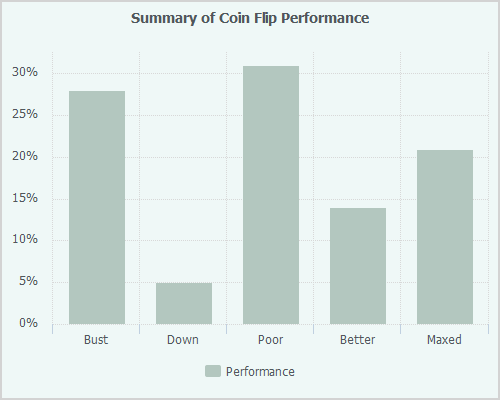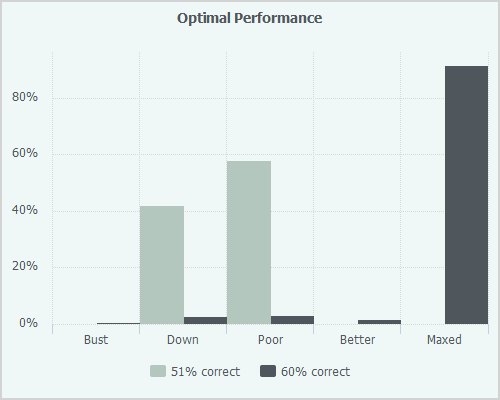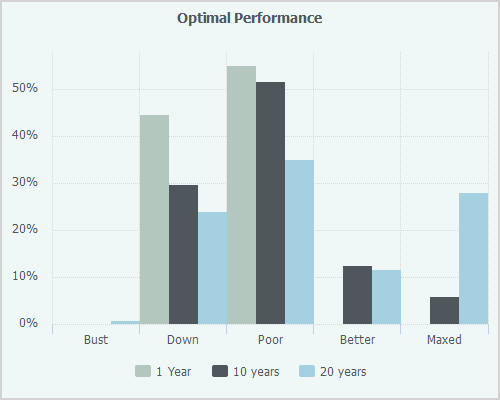# You Probably Can't Lose

#### 8 December 2016 / Dr Ewan Kirk, Dr Tom Gillam

What can an interesting and surprising experiment with finance students and finance professionals tell us about financial decisions and how to maximise extracting returns from low information content systems?

### Introduction

It is well known that humans are bad at estimating probabilities. We overestimate how likely very low probability events are and we get confused estimating the relative probabilities of events. Many of the well known behavioural biases in finance come from incorrect estimation of probabilities but even worse than this, it turns out that even when we know the probability distribution our use of probabilties is very poor indeed.

### The Evidence

A recent paper by Victor Haghani and Richard Dewey has received a lot of coverage in the press. Both the FT and Bloomberg wrote great articles about the experiment.

Haghani and Dewey constructed a coin flipping experiment. The participants were told that the coin they were flipping had a 60% chance of landing heads and a 40% chance of landing tails. They were given \$25 and told they could bet as much as they wanted on each toss and they could continue to play the game for 30 minutes and could toss the coin as often as they liked. The maximum payout for each participant was capped at \$250.

The 61 participants were either finance and economics students or young financial industry professionals. They were fully briefed on the game and, unlike so many psychology experiments, the experimenters told them the truth! You really were playing a game with a 60% chance of winning.

You can play the game yourself but before you do, take a minute and think about what your strategy should be.

Even without a deep grounding in probability theory, some rules seem fairly sensible.

• It makes sense to bet on heads most, if not all of the time
• Since the game is biased in your favour, it makes sense to play as often as you can
• It makes sense not to bet your entire stake on a single coin toss (because you’ve got a 40% chance of losing everything)
• Betting a small amount every time ($1 say) is unlikely to maximise winnings • If you’ve made money up to now then you can probably afford to bet a bit more These all seem like pretty sensible rules. If you’ve got 30 minutes to spare then give it a go…although you may save yourself time by reading the rest of this post first. ### What is the optimal strategy? Firstly, you should never bet on tails. Never ever ever ever. That should be pretty obvious. How much to bet is a little more complicated but not much more. With some fairly loose but sensible assumptions the optimal fraction$F^\star$of your wealth to bet is given by \begin{equation} F^\star = 2P - 1 \end{equation} where$P$is the probability that you win. This is the famous Kelly Criterion, which was originally formulated in 1956 and is very well known both in the gambling community and the finance community. This has all the properties that one would expect. If you have no information (it’s a 50/50 bet) then don’t bet. If you’re 100% sure then bet the farm. And as your wealth increases or decreases then bet more or less proportionally. ### How did the subjects do? Badly.In Figure 1 we have five, fairly arbitrary, categories: • “Bust” is less than \$2 at the end
• “Down” are those people who ended up with less than \$25 • “Poor” is between \$25 and \$100 • “Better” is between \$100 and \$250 • “Maxed” is hitting \$250

During the rest of this piece, we’re going to continue to use these groupings.

The big surprise is that so many people (17) went bust. These are educated financial professionals who managed to go completely bust in a game which unlike the “real” financial world, was simple, understandable and massively rigged in their favour. Nearly as surprising is that 18 of the subjects bet their entire bank roll on a single flip thus giving themselves a 40% chance of complete ruin.

The authors also note that despite an incredibly clear description of the probabilities, nearly half of the participants bet on tails more than 5 times, which is almost laughably suboptimal. If you have read any behavioural finance or psychology literature then experimenting with betting on tails once or twice may actually be a smart strategy. It’s always possible in these experiments that the researchers are actually investigating something else. Think of the infamous Milgram Experiment! I can imagine thinking “I wonder if something interesting will happen if I click on tails”. But five times?

This is a well designed and simple experiment which uncovers some very interesting issues. The authors of the paper conclude

“There is a meaningful gap in the education of young finance and economics students when it comes to the practical applications of the concepts of utility and risk taking.”

We would strongly support this conclusion and wholeheartedly support a better link between theory and practical applications in finance, statistics and computer science. Nevertheless, our purpose here is somewhat different: we want to look at the results of this experiment through a different lens. Do the results indicate a better way to invest now? The wheels of academia grind slowly and investors can’t wait for a new generation of better educated practitioners to arrive.

### How good is good?

Whilst a 60% chance of winning seems like quite a low probability, in the field of finance (and gambling) this is about as good as it gets. Blackjack players who can perfectly card count can expect a 2.5% edge on every hand. This is not quite equivalent to 52.5/47.5 odds due to the variable bet sizes but it’s a good approximation. A 2.5% financial upside probably doesn’t offset the downside of the casino taking you outside and breaking your legs when they discover you card counting…

As we have repeatedly said before, we humans are bad at estimating probabilities and also bad at estimating what constitutes a good performance. It would be very interesting to be able to estimate what realistic odds one can expect to have when you make a financial decision. A little bit of mathematics – which can be skipped without losing the thread of the argument – will allow us to translate from the world of binary bets with odds into the financial world of trading, volatilities and Sharpe ratios.

Let’s assume

• $P$ is the probability that your prediction of the direction of the market tomorrow is correct
• each return is independent
• a constant known market volatility of $\sigma$.

If we make the simplifying assumption that returns are $N(0, \sigma^2)$ then the expected return each day is

\begin{equation} E[r] = P E^{\texttt{+}}[r] + (1-P)E^{\texttt{-}}[r] \end{equation}

where $E^{\texttt{+}}$ is expectation over the positive returns and $E^{\texttt{-}}$ is the expectation over the negative returns. With the normality assumption then

\begin{equation} E^{\texttt{+}}[r] = -E^{\texttt{-}}[r] = \sigma\sqrt{\frac{2}{\pi}} \end{equation}

and therefore the expected return is

\begin{equation} E[r] = \sigma\sqrt{\frac{2}{\pi}}(2P-1) \end{equation}

To make this a little more concrete, let’s assume that we have an asset with a known and constant annualised volatility of 10%. On a daily basis the standard deviation of the returns is approximately 0.10/16, which is 62.5bp. From the above, the daily expected return if we are 60% correct in our call of the direction of the market tomorrow is

\begin{equation} E[r] = 0.00625\sqrt{\frac{2}{\pi}}(2 * 0.6 - 1) \approx 10\text{bp} \end{equation}

Therefore knowing the direction of the market move tomorrow with a 60% probability on average will make 10bp a day. Do this every day for a year and you’ve made 25.5% and your Sharpe ratio is around 2.5.

A Sharpe ratio of 2.5 on a single asset is exceptionally good. This is the stuff of dreams for systematic and disretionary managers. Given that this is exceptional (impossible?) we can conclude that the probability of a typical discretionary or systematic decision being correct is much smaller than 60%.

Most investment managers trade more than one asset in the hope of making Sharpe ratio gains through diversification. We have discussed this at length in a previous post and we have postulated that there are at most 10 uncorrelated “eigen-assets” which one can trade. For $N$ uncorrelated assets with a daily standard deviation of $\sigma$ and where the probability of choosing the correct direction for each asset is $P$ then the expected return of this portfolio is

\begin{equation} r = N\sigma\sqrt{\frac{2}{\pi}}(2P-1) \end{equation}

If we lower our sights and aim for a Sharpe ratio of 1 with a 10% volatility, we need a daily return of about 3.5bp. Rearranging the equation above, we find that the probability that each daily decision is correct is a bit less than 51%! Next time a manager discusses his or her positions with you, remember that the probability that they’re correct in their call is almost certainly less than 55% and probably less than 51%.

Whilst there are a significant number of simplifying assumptions in the toy model above, it does indicate that in a real financial setting, the probabilities of trades or decisions being correct are very close to 51/49. These are so close to being completely random that our minds are not well equipped to cope with the dominant randomness. The subjects of the Haghani and Dewey experiment had it easy. Once those students and young professionals start making real financial decisions, they are going to be dealing with an environment which is likely to be considerably more difficult to deal with than the one that many of them failed so spectacularly in.

### Scaling up

Let’s simulate how our subjects might have done in the real world. Let’s assume that we allow them to trade every business day in the year (256 days). We will assume that, chastened by the experience of the previous experiment, they have mugged up on the Kelly Criterion and they know that the optimal approach is always to bet in the direction of the low grade information that they have. Let’s look at the difference between 51% and 60%There are a couple of things to note in this result. If you do actually have a consistent 60/40 edge on your trades every day then firstly you have a 90% chance of maxing out and “winning” the game. Possibly surprisingly, you also have a very small chance of going bust at the end of the year.

But, remember we are extremely unlikely to have a 60% hit rate. How long do we need to give the players with the 51% hit rate until most of them do “well”?With a 51% probability of being correct, even after 10 years, there are still around a quarter of the “participants” who are down and there are very few people who have maxed out the game.

### Conclusion

So what is the solution to this problem?

The paper by Haghani and Dewey shows that investors make poor decisions even when the probability of winning on a position is 60%. What we have shown is that the typical probability of an individual position being correct (in sign) is not much larger than 50%. We have also shown that for single positions you need decades of using this typically small edge before the “edge” manifests itself. Very few managers or allocators have the luxury of multi-decade investment horizons.

If many repeated uses of small edges over time is impractical, what else can we do? Our old friend diversification comes to the rescue here. If we can take multiple independent bets each day then we would expect to achieve better results. Furthermore, the more independent bets we can take, the better. This leads us to a view that systematic investing across many decisions and independent assets is by far the most effective and efficient way to invest when the signals are weak.

But how can an investor research, understand and evaluate so many positions every day? If our investor were an equity investor, ideally she would have a considered view on 1000, 2000 or better still 15,000 stocks and be able to leverage her low probability of being correct on each one into significant alpha. However, this is practically impossible. Evaluating 15,000 stocks daily would give our imaginary manager approximately 2 seconds to make a decision on each one every day.

Consequently in reality our manager screens a far smaller universe and has narrow and concentrated positions in which she has high conviction. Obviously if her edge is high (say 60%), then this is can be profitable for her investors – and let us be clear, some discretionary managers do have significant edge in niche markets or unloved and neglected stocks – but in the domain of large and midcap stocks (where the majority of all the world’s equity investments are), the edges are and will be small.

However, help is at hand. Computers can evaluate an entire 15,000 stock universe easily. They can perform a rigourous and disciplined analysis of each stock on hundreds of dimensions and come up with an estimate of the probability of outperformance or underperformance and can do this in milliseconds. They can then apply the same technique across thousands of stocks and combine the individual positions in optimal ways accounting for volatilities, correlations, liquidity and trading costs. In this way, small signals run on thousands of positions can be multiplied up into attractive returns very efficiently.

Whether it is in the domain of macro trading or single stock trading, it is likely that as time passes, the available “edge” in markets will decrease. But, unless markets become perfectly efficient random noise, the edge will not end up being zero but will become smaller. This will favour those who can combine as many of these 51/49 (or even 50.1/49.9) indicators together efficiently and effectively.

The rise of the machines in investing will continue.